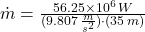Question

Suppose the dam is 80% efficient at converting the water’s potential energy to electrical energy. How many kilograms of water must pass through the turbines each second to generate 45.0 MWMW of electricity? This is a typical value for a small hydroelectric dam.

1.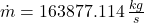Explanation:

Let assume that the water dam has a fall of 35 meters. The required instantaneous potential energy is: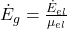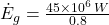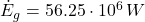The instantaneous potential energy has the following model: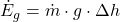The mass flow rate needed to produced the required energy production: